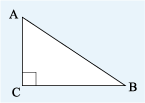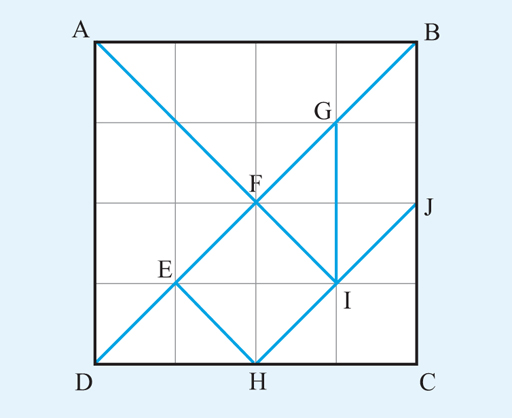Science, Maths & Technology

### Become an OU studentSucceed with maths: part 2

Start this free course now. Just create an account and sign in. Enrol and complete the course for a free statement of participation or digital badge if available.

# 1.4 Describing shapes

When you are describing a geometrical figure or shape, you often need to refer to a particular line or angle on the diagram, so others know what you are referring to. This can be done by labelling the diagram with letters. For example, Figure 10 shows a triangle labelled clockwise at the corners with A,B,C. This is then known as triangle ABC, in which the longest side is AB and the angle is a right angle. is the angle formed by the lines AC and CB. The point where two lines meet is known as a vertex (the plural is vertices). So A, B and C are vertices of the triangle.Figure 10 A triangle labelled ABC

Note that you can use the shorthand notation ‘’ for ‘the triangle ABC’ if you wish. There is a lot of new maths vocabulary in these last few sections, so you might find it useful to make a note of these to refer back to when completing this next activity, or for this week’s quiz and the badged quiz in Week 8.

## Activity 1 What can you see?

Timing: Allow approximately 10 minutes

Look at the image below and then answer the following questions using the letters shown.Figure 11
• a.Which sets of lines appear to be parellel?

• a.
• b.AB is parallel to DC.

AD is parallel to GI and BC.

AI is parallel to EH.

BD is parallel to HJ

• b.Which lines are perpendicular?

• b.AB is perpendicular to AD and to BC.

DC is perpendicular to AD and BC.

EH is perpendicular to DB and HJ.

AI is perpendicular to HJ and DB.

• c.How many triangles can you see?

• c.
• d.What other shapes can you see?